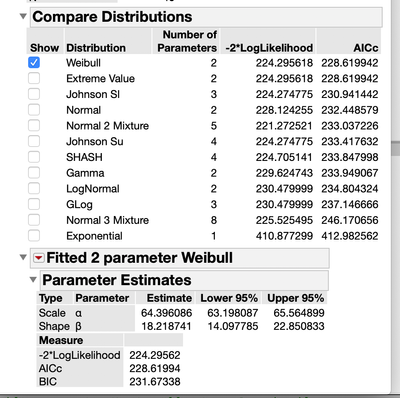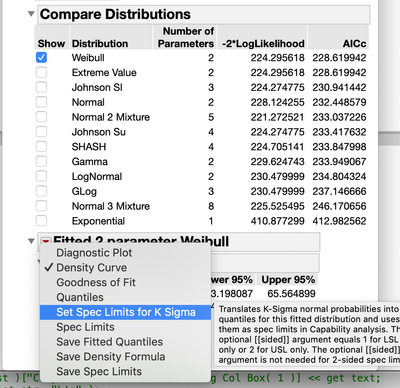Choose Language Hide Translation Bar
Highlighted

## Distribution Platform: Difficulty Using "Set Spec Limits for K Sigma" when all fits are used

Hi.  In the Distribution platform, is there a way to refer back to a fitted distribution if the Fit Distribution("All") option is used?

Context: I have a script that fits multiple distributions to my data using the Distribution platform.  When I include the Fit Distribution("All") option, the report includes a table "Compare Distributions" with the ranking of each distribution.  For example:

``````Names Default To Here(1);
dt = Open("\$SAMPLE_DATA/Big Class.jmp");

dist = Distribution(
Continuous Distribution(
Column(dt:Height),
Quantiles(0),
Vertical(0),
Outlier Box Plot(0),
Fit Distribution("All")
)
);
dist << (Fit Handle << Set Spec Limits for K Sigma(3));	//Does nothing``````

My problem occurs when I want to use the "Set Spec Limits for K Sigma" command.  Naturally I must indicate which one of the specific fits this is meant for.  I've tried using Fit HandleI, intending to refer to the first fit in the "Compare Distributions" table, but this has no effect.

In the example above, I can use Set Spec Limits for K Sigma command in the GUI (i.e. report window), so in theory I believe I should be able to do this programmatically as well.  So far the best option I can think of is to use the above code to identify the best distribution, the re-perform a separate fit using that specific distrubtion, which I would then be able to refer the Set Spec Limits for K Sigma command to.  This involves extra computation and code though, which I would prefer to avoid.

Any help is appreciated.  Thanks.

3 REPLIES 3
Highlighted

## Re: Distribution Platform: Difficulty Using "Set Spec Limits for K Sigma" when all fits are used

I, also, was not able to get the Fit Handle to work, but I did find a work around.  Hopefully, you will not have to use my work around, because another Community Member will provide the Fit Handle code

``````Names Default To Here( 1 );
dt = Open( "\$SAMPLE_DATA/Big Class.jmp" );

dist = Distribution(
Continuous Distribution(
Column( dt:Height ),
Quantiles( 0 ),
Vertical( 0 ),
Outlier Box Plot( 0 ),
Fit Distribution( "All" )
)
);

theDist = Trim( Word( 3, Report( dist )["Compare Distributions"][String Col Box( 1 )] << get text, "\!n" ) );
Eval(
Substitute(
Expr(
dist << Fit Distribution( __dist__( Set Spec Limits for K Sigma( 3 ) ) )
),
Expr( __dist__ ), Parse( theDist )
)
);``````
Jim
Highlighted

## Re: Distribution Platform: Difficulty Using "Set Spec Limits for K Sigma" when all fits are used

Hi Tx.  Thanks for replying to this.  I see your solution finds the best distribution from the "Compare Distributions" table in the report, and then creates a new, separate fit using the Fit Distribution() command, to which a Set Spec Lims command can be sent.  Unfortunately this is what I'm trying to avoid since it involves computing the best fit distribution twice (once with the Fit Distribution(All) command, and again with the Fit Distribution(theDist) command).  That being said, unless there's a better option (sigh), this seems to be how it must be done.

An alternate idea occured to me...

In the GUI, one can click on the red down arrow next to the distribution and select "Set Spec Limits...", as shown below.  Do you suppose there's a way to tell JSL to "click" on the RDA and select the 5th item in the resulting dropdown list?  That is, rather than figure out the commands to do this, just have JSL hijack the GUI.  Is anything like that possible?Thanks again for the help.

Highlighted

## Re: Distribution Platform: Difficulty Using "Set Spec Limits for K Sigma" when all fits are used

You should use Fit Handle["Best"] .

``````dt = Open( "\$SAMPLE_DATA/Big Class.jmp" );

dist = Distribution(
Continuous Distribution(
Column( dt:Height ),
Quantiles( 0 ),
Vertical( 0 ),
Outlier Box Plot( 0 ),
Fit All
)
);

dist << (Fit Handle["Best"]  << Process Capability(Set Sigma Multiplier for Quantile Spec Limits(3)));
``````
Article Labels

There are no labels assigned to this post.